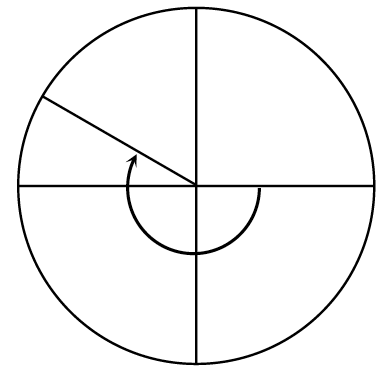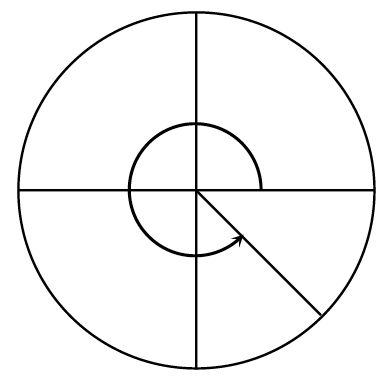### Home > PC3 > Chapter 4 > Lesson 4.1.2 > Problem4-32

4-32.

Below are two of the special angles that are used in a unit circle. Identify the radian measure for the angle shown, then state the sine, cosine, and tangent of the angle. Remember, counterclockwise angles are positive and clockwise angles are negative.

Divide each circle into an equal number of parts. Remember that half of the circle is $\pi$ radians.

1.1.